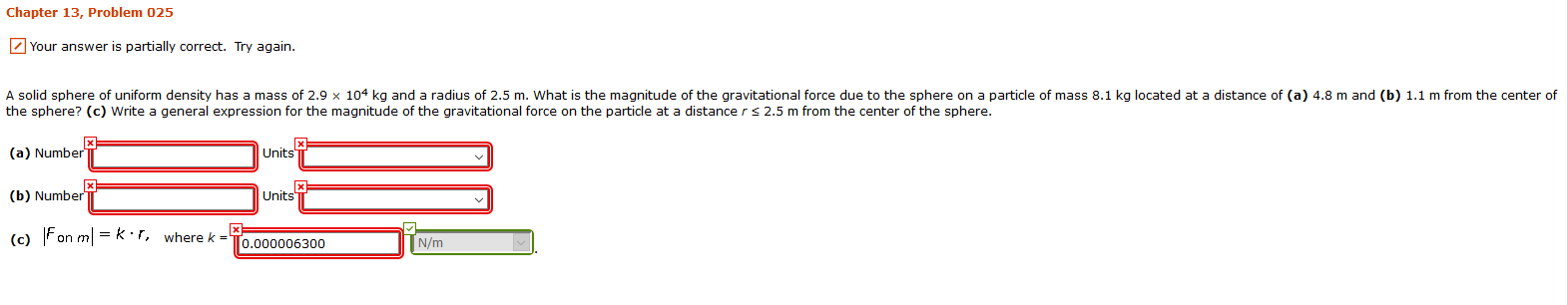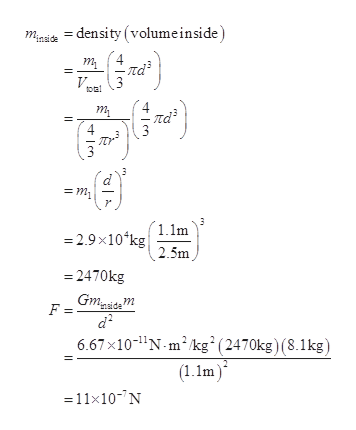# Chapter 13, Problem 025Your answer is partially correct. Try again.A solid sphere of uniform density has a mass of 2.9 x 104 kq and a radius of 2.5 m. What is the magnitude of the gravitational force due to the sphere on a particle of mass 8.1 kg located at a distance of (a) 4.8 m and (b) 1.1 m from the center ofthe sphere? (c) Write a general expression for the magnitude of the gravitational force on the particle at a distance rs 2.5 m from the center of the sphere.(a) NumberUnits(b) NumberUnits(c) Fon mk r, where k0.000006300N/m

Question
2 viewshelp_outlineImage TranscriptioncloseChapter 13, Problem 025 Your answer is partially correct. Try again. A solid sphere of uniform density has a mass of 2.9 x 104 kq and a radius of 2.5 m. What is the magnitude of the gravitational force due to the sphere on a particle of mass 8.1 kg located at a distance of (a) 4.8 m and (b) 1.1 m from the center of the sphere? (c) Write a general expression for the magnitude of the gravitational force on the particle at a distance rs 2.5 m from the center of the sphere. (a) Number Units (b) Number Units (c) Fon mk r, where k 0.000006300 N/m fullscreen
check_circle

Step 1

Given,

Mass of the sphere m1=2.9×104kg

Mass of the particle m2=8.1kg

Step 2

a)

Calculate the gravitational force at a distance d=4.8m from the center of sphere.(d>r)

Step 3

b)

The gravitational force at a distance 1.1m from...help_outlineImage Transcriptionclosemasi =density (volumeinside V toal 3 т t лаз 3 1.1m -2.9x10 kg 2.5m 2470kg Gmgice m F = - 6.67x10-1N-m2kg2(2470kg) (8.1kg) (1.1m =11x10- N fullscreen

### Want to see the full answer?

See Solution

#### Want to see this answer and more?

Solutions are written by subject experts who are available 24/7. Questions are typically answered within 1 hour.*

See Solution
*Response times may vary by subject and question.
Tagged in

### Gravitation